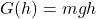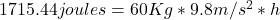## The label on a candy bar says 410 Calories. For the steps and strategies involved in solving a similar problem, you may view a Video Tutor S

Question

The label on a candy bar says 410 Calories. For the steps and strategies involved in solving a similar problem, you may view a Video Tutor Solution. Part A Assuming a typical efficiency for energy use by the body, if a 60 kg person were to use the energy in this candy bar to climb stairs, how high could she go?

in progress 0
2 months 2021-07-30T01:22:37+00:00 2 Answers 5 views 0

h = 729.66 m

Explanation:

We are given;

Energy contained in candy bar;

Q_in = 410 calories = 410 x 4190 J =

1,717,900 J

Now the formula for efficiency of a human body is;

e = work output /work input = Q_out/Q_in

The efficiency of a human body is 0.25. Thus;

0.25 = Q_out/Q_in

So, Q_out = 0.25 x 1,717,900

Q_out = 429,475 J

This Q_out is the gravitational potential energy

Thus, we know that the formula foe Potential energy is;

PE = mgh

Where m is mass, g is acceleration due to gravity and h is height.

Thus,

429,475 = 60 x 9.81 x h

h = 429,475/(60 x 9.81)

h = 729.66 m

2.9174meters.

Explanation:

410Calories = 1715.44 Joules (Energy).

we have essentially calculate height at which Gravitational potential is 1715.44 Joules.

We know that Gravitational Potential as a function of height above reference point is..

Substituting values in gives.in above solve for h and you will get.

h = 2.917meters (units are meters by dimentional analysis ).

That is the height a 60Kg person can reach by climbing stairs.